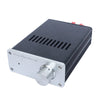\$61.19 \$81.59
Saved 25% (\$20.40)
Quantity

Sale ends once the timer hits zero!

0
0

Hours

0
0

Minutes

0
0

SecondsShare:
Description
Shipping & Return
Our Guarantee
Review

0

Specifications:

DC input port size 5.5MMx2.1MM

DC=50V,R=4Ω,THD+N=10%,P=300W
DC=50V,R=6Ω,THD+N=10%,P=210W
DC=50V,R=8Ω,THD+N=10%,P=160W

DC=50V,R=4Ω,THD+N=1%,P=240W
DC=50V,R=6Ω,THD+N=1%,P=160W
DC=50V,R=8Ω,THD+N=1%,P=125W

Total harmonic distortion (THD+N): 1%
Signal to noise ratio (SNR):>100dB
Input impedance: 20K
Output offset voltage: 150mV